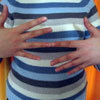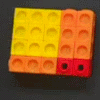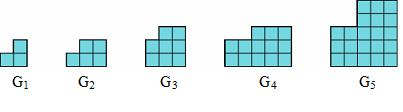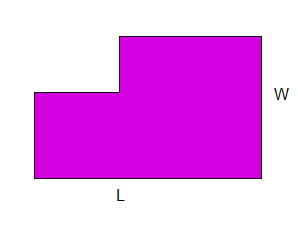#### You may also like### Building Gnomons

Build gnomons that are related to the Fibonacci sequence and try to explain why this is possible.### What Is the Question?

These pictures and answers leave the viewer with the problem "What is the Question". Can you give the question and how the answer follows?Can you find out what is special about the dimensions of rectangles you can make with squares, sticks and units?

# Gnomon Dimensions

##### Age 14 to 16Challenge Level

You may wish to try the related problem Building Gnomons first.

A Gnomon is a rectangle with another rectangle cut out of one corner. The area of each Gnomon is a Fibonacci number. (The Fibonacci numbers are $1, 1, 2, 3, 5, 8$ and so on, with each new term being the sum of the previous two terms.)$G_1$ has area $3$, $G_2$ has area $5$, $G_3$ has area $8$ and so on.

Draw the next three gnomons in the sequence.

Look at the length and width of the large rectangle from which each gnomon is made.Is there a pattern to the lengths and widths?
Can you generalise?

Now look at the length and width of the rectangle cut out of each gnomon. Can you see any patterns here? Can you generalise and justify what you see?

I want to group the gnomons with area 3, 8 and 21 together, and the gnomons with area 5, 13 and 34 together. Can you explain why I want to divide these into two separate groups?

Can you give a convincing argument why all the gnomons fit into one of these two groups?

What other interesting patterns and relationships can you find?